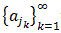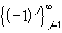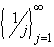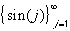#Interactive Real Analysis

## 3. Sequences of Numbers

### 3.3. Subsequences

So far we have learned the basic definitions of a sequence (a function from the natural numbers to the Reals), the concept of convergence, and we have extended that concept to one which does not pre-suppose the unknown limit of a sequence (Cauchy sequence).

Unfortunately, however, not all sequences converge. We will now introduce some techniques for dealing with those sequences. The first is to change the sequence into a convergent one (extract subsequences) and the second is to modify our concept of limit (lim sup and lim inf).

 Definition 3.3.1: Subsequence Letbe a sequence. When we extract from this sequence only certain elements and drop the remaining ones we obtain a new sequences consisting of an infinite subset of the original sequence. That sequence is called a subsequence and denoted byOne can extract infinitely many subsequences from any given sequence.
 Examples 3.3.2:Take the sequence, which we have proved does not converge. Extract every other member, starting with the first. Does this sequence converge ? What if we extract every other member, starting with the second. What do you get in this case ?Take the sequence. Extract three different subsequences of your choice. Do these subsequences converge ? Is so, to what limit ?
The last example is an indication of a general result:
 Proposition 3.3.3: Subsequences from Convergent Sequence Ifis a convergent sequence, then every subsequence of that sequence converges to the same limit Ifis a sequence such that every possible subsequence extracted from that sequences converge to the same limit, then the original sequence also converges to that limit.

The next statement is probably one on the most fundamental results of basic real analysis, and generalizes the above proposition. It also explains why subsequences can be useful, even if the original sequence does not converge.

 Theorem 3.3.4: Bolzano-Weierstrass Letbe a sequence of real numbers that is bounded. Then there exists a subsequencethat converges.
 Example 3.3.5:Doesconverge ? Does there exist a convergent subsequence ? What is that subsequence ?In fact, the following is true: given any number L between -1 and 1, it is possible to extract a subsequence from the sequencethat converges to L. This is difficult to prove.
Next, we will broaden our concept of limits.